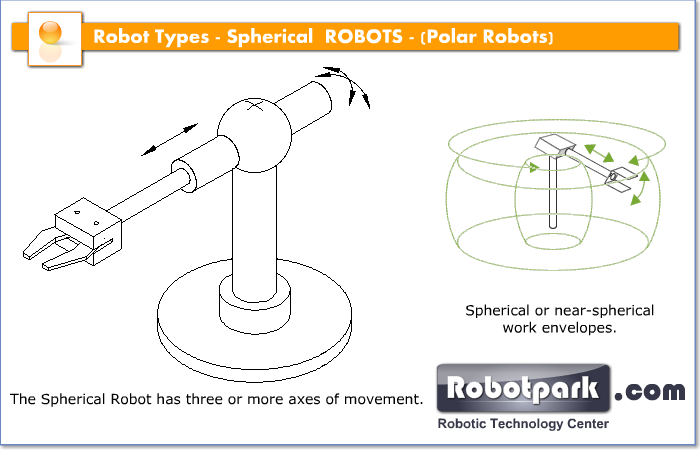## SPHERICAL ROBOTS

Spherical Coordinate Robots

spherical robot is a robot with two rotary joints and one prismatic joint; in other words, two rotary axes and one linear axis. Spherical robots have an arm which forms a spherical coordinate system.Spherical Coordinate System:

In mathematics, a spherical coordinate system is a coordinate system for three-dimensional space where the position of a point is specified by three numbers: the radial distance of that point from a fixed origin, its polar angle measured from a fixed zenith direction, and the azimuth angle of its orthogonal projection on a reference plane that passes through the origin and is orthogonal to the zenith, measured from a fixed reference direction on that plane.

The radial distance is also called the radius or radial coordinate. The polar angle may be called colatitude, zenith angle, normal angle, or inclination angle.